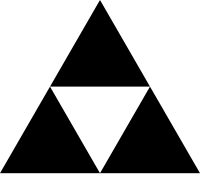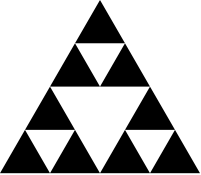Review questions-1

What is the output value and data type of the following expression?

    (/ (+ 2 3) (* 2 3))

Review questions-2

What is the output value and data type of the following expression?

    (and (< 2 3) (> 2 3))

Review questions-3

What is the output value and data type of the following expression?

    (image-width (square 100 "solid" "black"))

Review questions-4

What is the output value and data type of the following expression?

    (scale 2 (square 100 "solid" "black"))

Review questions-5

What is the output value and data type of the following expressions?

    (define a 2)
(define b 4)
(cond
((> a b) "a")
((< a b) "b")
(else    "n"))

Review questions-6

What is/are the mistake(s) in the following program?

    (define (f x)
(+ x y))

Review questions-7

What is/are the mistake(s) in the following program?

    (define (f x y)
(cond
((+ x y) x)
(else    y)))
(f 3 4)

Review questions-8

What is/are the mistake(s) in the following program?

    (define (f x y)
(cond
((< x y) x)
(else    y)))
(f "a" "b")

Case studies with functions and conditionals

Below are some basic questions on function design.

Case: difference

Write a function to return the absolute difference of two given numbers.

Case: Overlay two shapes

Write a function to return an overlay of two given shapes, depending on which one is wider, put the narrow one on top of the wider one. You can use image-width function.

Case studies with recursion

Recursion may be useful in a variety of scenarios, and hence used in a variety of ways. Here we examine some generic use cases.

Case: formulating problems

Consider the following infinite sum: $s_{\infty}(n,m)= \frac{n}{m}+\frac{n^2}{m^2}+ \frac{n^3}{m^3} +\cdots$

Produce a recursive formulation of the function above.

Solution:

$s_{\infty}(n,m)= \frac{n}{m}+\frac{n^2}{m^2}+ \frac{n^3}{m^3} +\cdots$ can be converted to: $s_{\infty}(n,m)= \frac{n}{m}\cdot (1 +\frac{n}{m}+\frac{n^2}{m^2}+ \frac{n^3}{m^3} +\cdots)$

Now we see that the value appears on the right hand side: $s_{\infty}(n,m)= \frac{n}{m}\cdot (1 + s_{\infty}(n,m))$ indeed one does not even need a recursive computation to find the result! $s_{\infty}(n,m) \cdot (1-\frac{n}{m})= \frac{n}{m}$ $s_{\infty}(n,m) \cdot = \frac{\frac{n}{m}}{(1-\frac{n}{m})}$

Case: is a number prime?

A number is prime if no number divides it except 1 and itself. To test if a number $$p$$ is prime we can take its modulo of all numbers starting with 2 up to $$p-1$$, and none of these modulos are 0 then we can say that the number is prime.

Formulate the problem in a recursive way, and write the program to compute it.

Solution: formulation

We face the same problem again: we do not know how many modulo trials we need to make, it depends on the value of the input given, i.e. the number $$p$$. We can however convert this control to a recursive formulation. Let us define a helper function which tells if a number is divisible by numbers up to some other number, $$d(p,k)$$, its value is true if any number from 2 to $$k$$ divides $$p$$. We can write this function in a recursive manner as follows: $d(p,k)=\left\{ \begin{array}{cc} false & \textrm{if k \le 1} \\ true & \textrm{if k\ge 2 and p \mod k = 0}\\ d(p,k-1) & \textrm{otherwise} \end{array}\right.$

Therefore primality test is converted into the following $isPrime(p)=! d(p,p-1)$

Solution

This is a kind of problem in which we know -at most- how many repetitions (i.e. iterations) will be done during recursion. To check primality of $$p$$ we recurse at most $$p-2$$ many times.

    (define (divisible p k)
(cond
((<= k 1) false)
((= (modulo p k) 0) true)
(else (divisible p (- k 1)))))
(check-expect (divisible 3 2) false)
(check-expect (divisible 12 11) true)
(define (isPrime p)
(not (divisible p (- p 1))))
(check-expect (isPrime 1) true)
(check-expect (isPrime 2) true)
(check-expect (isPrime 4) false)
(check-expect (isPrime 17) true)
(check-expect (isPrime 25) false)

Case: finding powers

Finding an integer power of a number, $$x^n$$ seems to require $$n-1$$ multiplications at first sight. However, this computation can be simplified greatly. Think about $$2^8$$, which would require 7 multiplications to compute. since the exponent 8 is an even number, this can be written as $$2^8={2^4}^2$$. Therefore we need 3 multiplications to compute $$2^4$$ and another multiplication to compute its square. It can be further simplified as $$2^8={2^4}^2={{2^2}^2}^2$$, which now requires only 3 multiplications. In case of an odd exponent, one can rewrite only for the even part: $$2^9=2^8\cdot 2$$.

Solution: formulation

A recursive formulation is: $x^n=\left\{ \begin{array}{cc} 1/(x^n) & \textrm{if n < 0} \\ 1 & \textrm{if n =0} \\ x & \textrm{if n =1} \\ (x^{n/2})^2 & \textrm{if n \mod 2=0} \\ (x^{(n-1)/2})^2\cdot x & \textrm{if n \mod 2\ne 0} \end{array}\right.$

Solution

    (define (power x n)
(cond
((not (integer? n)) (error "exponent not integer"))
((< n 0) (/ 1 (power x (- n))))
((= n 0) 1)
((= n 1) x)
((= n 2) (* x x))
((even? n) (power (power x (/ n 2)) 2))
(else (* x (power (power x (/ (- n 1) 2)) 2)))))
(power 2 32)
(power 2 -2)

Case: repeating shapes

In this problem we wish to overlay a smaller version of a shape onto itself, and repeat this process until a minimum size is reached. For example we will overlay a 10% smaller version of a circle onto itself, and further %10 scaled version on top, etc., until the size of circle reaches 20, where all these numbers and the shape itself is given as parameters to the function. Remember that size of an image can be found using ımage-height or image-width functions.

Solution

This is also a situation in which we know the number of recursions, at least implicityly.

    (define (repeatingShapes shape scaleFactor limit)
(cond
((and (<= (image-width shape) limit) (<= (image-height shape) limit)) shape)
(else (overlay
(repeatingShapes (scale scaleFactor shape) scaleFactor limit)
shape))))
(repeatingShapes (circle 100 "outline" "black") 0.9 20)
(repeatingShapes (square 100 "outline" "black") 0.9 20)

Case: Sierpinski fractal

A fractal is a recursive shape. The recursion pattern is a simple replacement pattern. An example is a Sierpinski fractal, whose replacement pattern is given below:In drawing a fractal we are given the overall size of the fractal, and a limit size. Until the limit size is reached we keep replacing parts of fractal with fractal pattern of the size of the part. In the pattern above, each triangle is replaced with a smaller version of the pattern. This replacement (i.e. recursion is continued until limit size is reached, in which case simply a triangle is placed). The pattern can be realized using above and beside commands.

In the case of Sierpinski, a repetition depth, instead of size limit is given.

Solution

This is also a situation in which we know the number of recursions. The recursion depth is $$n$$, and it is a three-fold recursion. So total number of calls is approximately (i.e. at the order of) $$3^n$$.

    (require 2htdp/image)
(define (sierpinski n)
(cond
((= n 0) (above
(triangle 10 "solid" "black")
(beside
(triangle 10 "solid" "black")
(triangle 10 "solid" "black"))))
(else  (above
(sierpinski (- n 1))
(beside
(sierpinski (- n 1))
(sierpinski (- n 1)))))))

(sierpinski 5)

Case Newton-Raphson roots

Newton's method is a way to find a root of any continuos and convex function, e.g. to find an $$x$$ where $$f(x)=0$$. The method starts of with some guess, $$x_i$$ and in each iteration the guess is improved unless $$-\epsilon < f(x_i) < \epsilon$$, where $$\epsilon$$ is a small sensitivity limit. Improvement is done by intersecting the slope at guess with x-axis. The initial guess, $$x_0$$ is usually taken as 1.

$x_{i+1}=x_i - \frac{f(x_i)}{f'(x_i)}$

When $$f(x)=x^n-A$$ what we find x as root $$f(x)=0$$ is $$x^n=A$$, ie. nth root of $$A$$. Since $$f'(x)=n\dot x^{n-1}$$, the iteration becomes $x_{i+1}=x_i - \frac{x_i^n-A}{n\cdot x^{n-1}}$

solution

This is a problem in which we cannot possibly know the number of repetitions.

    (define (root a n)
(Newton a n 1.0 0.01))

(define (Newton a n xi e)
(cond
((< (abs (- (expt xi n) a)) e) xi)
(else (Newton a n (- xi (/ (- (expt xi n) a) (* n (expt xi (- n 1))))) e))))

(root 2 2)
(root 27 3)